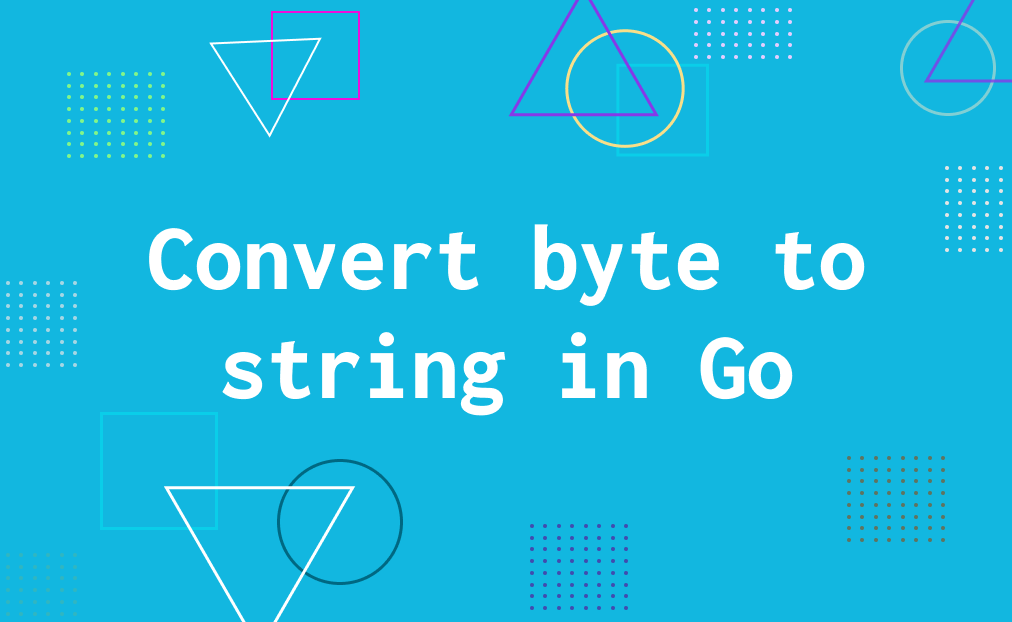# How to convert byte to string in Golang?

When working with golang you will most likely have scenario when you need to convert `byte` slice to `string`. There's several way to do this, let's explore how we can convert byte to string or string to byte in golang.

## Primitive string function

This is the most easy way to convert byte to string. And it's okay it works and it standard way to do it.

``````b :=[]byte("example")
result := string(b)
``````

But there's some issue with this method, which is the process of this method is slow.

## Using unsafe pointer

If you want the process of this became fast let use this method.

``````import "unsafe"

func ByteToString(b []byte) string {
return *(*string)(unsafe.Pointer(&b))
}
``````

## Compare

So let's compare this method.

``````func ByteToString(b []byte) string {
return *(*string)(unsafe.Pointer(&b))
}

func ByteToString2(b []byte) string {
return string(b)
}

func main() {
start := time.Now()
b := []byte("€xample 💡")
for i := 0; i < 10000000; i++ {
ByteToString(b)
}
end := time.Now()
fmt.Printf("unsafe.Pointer(&b) : %+v / 10000000 operation\n", (end.Sub(start)))

start = time.Now()
for i := 0; i < 10000000; i++ {
ByteToString2(b)
}
end = time.Now()
fmt.Printf("string(b) : %+v / 10000000 operation\n", (end.Sub(start)))
}
``````

So the result would be like this.

``````unsafe.Pointer(&b)  : 2.9967ms / 10000000 operation
string(b)           : 53.9999ms / 10000000 operation
``````
Have any question? Connect with me in twitter